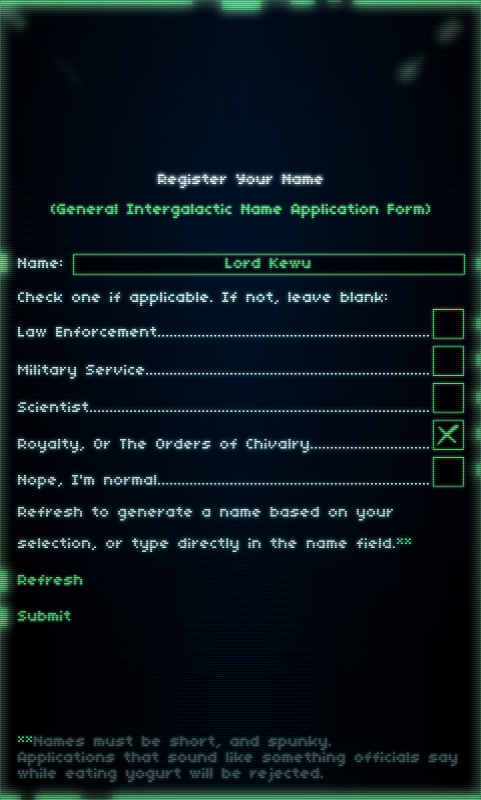The Wacky Name Generator (Version 1.0.0.0.1.0.2)
Try it bellow:

So I’m making a game!
The premise is that you play a monster tearing up a fictional city. Any city really.
Probably Tokyo, but I’m undecided.
The other great premise is that you eat A LOT of civilians, military, and law enforcement officials.
To add a touch of random (and reduce the time it takes for coming up with names for an “expendable” population), names for each enemy sprite are randomly created (in this case pieced together using a set amount of vowels and consonants), and then applied to the sprite.
It’s intended for cute little dinky Asian sounding short names (first + last + occasional suffix).

Some made me laugh so I figure good use could be had from playing with a name generator version of the code.

```//USAGE:
//trace(generate_name(2,0,arr_prefix,arr_suffix));
//trace(generate_name(2,2,arr_prefix,arr_suffix));
```

Code:

```//letters (vowels and consonants) for the names
var arr_vowels:Array = new Array("A","E","I","O","U");
var arr_cons:Array = new Array("B","C","D","F","G","H","J","K","L","M","N","P","Q","R","S","T","V","W","X","Y","Z");
//suffixes (applying a suffix is random)
var arr_suffix_city:Array = new Array("B.A.", "B.F.A.", "B.Tech.", "B.Sc.", "M.A.", "M.F.A.", "LL.M", "M.L.A.", "M.B.A.", "M.Sc.", "J.D.", "M.D.", "D.O.", "Pharm.D.", "Ph.D.", "D.Phil.", "LL.D", "Eng.D.");
var arr_suffix_civilian:Array = new Array("Esq.", "Esquire", "Esq.", "esq.", "Jr.", "Junior", "Jnr.", "Snr.");
//prefixes
var arr_prefix_law:Array = new Array("Detective", "Inspector", "Investigator", "Captain", "Lieutenant", "Sergeant","Inspector", "Commander", "Colonel", "Undersheriff", "Deputy", "Chief", "Commissioner", "Superintendent", "Sheriff");
var arr_prefix_civilian:Array = new Array("Mr.","Mrs.","Mister","Miss");
//the currently selected prefix and suffix
var arr_suffix:Array= new Array();
var arr_prefix:Array= new Array();
//above set to a default (on first run)
arr_suffix = arr_suffix_civilian;
arr_prefix = arr_prefix_civilian;
//to length of the text fields... otherwise just pass it a hard coded value
var num_fnLength:Number = int(txt_fncnt.text);
var num_lnLength:Number = int(txt_lncnt.text);
//
function generate_name(fnLength:Number, lnLength:Number, arrPrefix:Array, arrSuffix:Array)
{
//prefix and suffix part
var randNumSuffix:Number = Math.ceil(Math.random()*100);//if bellow a certain number then yes show one
var randSuffix:String = arrSuffix[Math.ceil(Math.random()*arrSuffix.length)-1];
var randPrefix:String = arrPrefix[Math.ceil(Math.random()*arrPrefix.length)-1];
//the name part
var firstName:String = "";
var lastName:String = "";
var fullName:String= "";//this will be returned;
//regexp to strip out any spaces left
//if user doesn't want last/first name (fnLength or lnLength are 0)
var reg_trimSpaces:RegExp = /^\s+|\s+\$/g;
//randomize the vowel vs. consonant order (so the name starts with either or)
var arr_firstNameOrder:Array = new Array(arr_vowels,arr_cons);
var arr_lastNameOrder:Array = new Array(arr_vowels,arr_cons);
arr_firstNameOrder = arr_firstNameOrder.sort(function(){return Math.round(Math.random());});
arr_lastNameOrder = arr_firstNameOrder.sort(function(){return Math.round(Math.random());});
//first name
for (var i:Number=0; i<fnlength ; ++i)
{
firstName +=  arr_firstNameOrder[Math.ceil(Math.random() * arr_firstNameOrder.length) - 1] + arr_firstNameOrder[Math.ceil(Math.random() * arr_firstNameOrder.length) - 1];
}
//last name
for (var j:Number=0; j<lnLength; ++j){
lastName +=  arr_lastNameOrder[Math.ceil(Math.random() * arr_lastNameOrder.length) - 1] + arr_lastNameOrder[Math.ceil(Math.random() * arr_lastNameOrder.length) - 1];
}
//edit so that ONLY the first letter of both names is uppercase (rest is lowercase)
firstName = firstName.substr(0, 1).toUpperCase()+firstName.substr(1, firstName.length).toLowerCase();
lastName = lastName.substr(0, 1).toUpperCase()+lastName.substr(1, lastName.length).toLowerCase();
//if it should have a suffix (and append a suffix)
if(randNumSuffix&lt;30){
fullName = randPrefix+" "+firstName+" "+lastName+" "+randSuffix;
}else{
fullName = randPrefix+" "+firstName+" "+lastName;
}
fullName = fullName.replace(reg_trimSpaces, "");//strip out whitespaces
//
return fullName;
}
```

Here is a screenshot of what it will be used for:Tetrageddon’s upcoming release released Offender 2 (World War B: War on Rabbits).
The Intergalactic Foundation for Non-Anthropomorphic Rights name registration form.

Enjoy!

Update:

And for those that are interested, here’s a Javascript version of the above (By David Galloway). Click here.